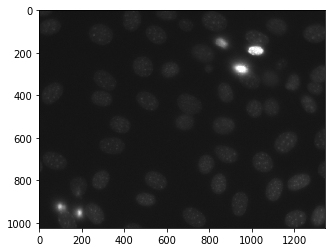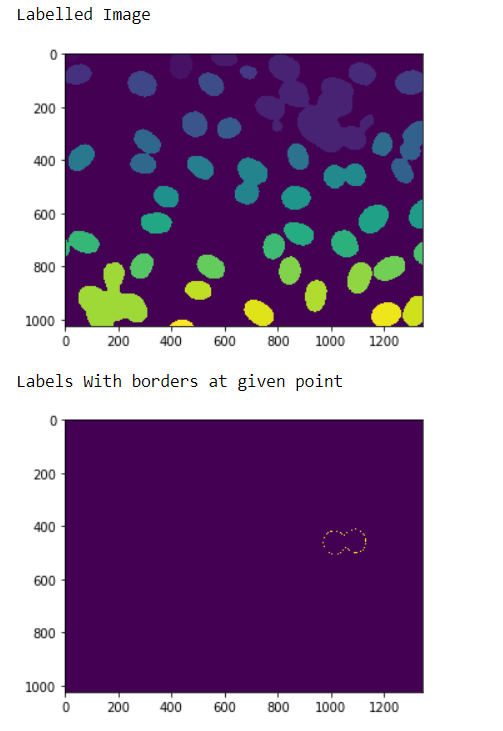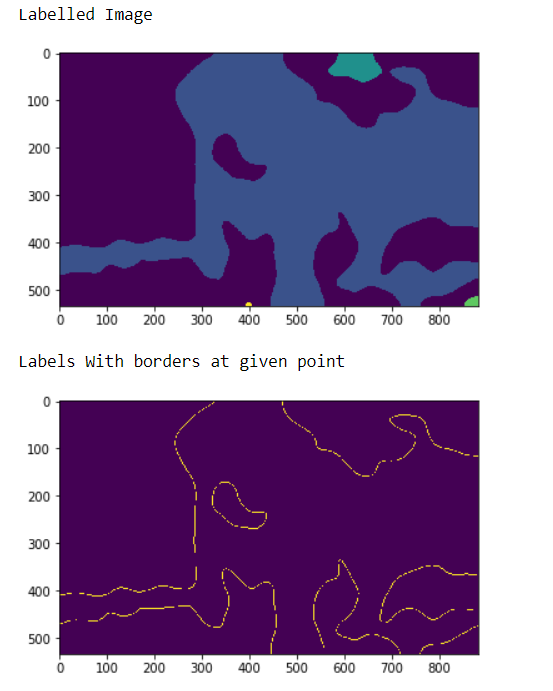# Mahotas – Getting Border of label at given region

• Last Updated : 13 Sep, 2022

In this article we will see how we can get the border of the label in the labelled image within the given region in mahotas. For this we are going to use the fluorescent microscopy image from a nuclear segmentation benchmark. We can get the image with the help of command given below

`mhotas.demos.nuclear_image()`

Below is the nuclear_imageIn order to do this we will use mahotas.labelled.border method

Syntax : mahotas.labelled.border(labelled_image, i, j)
Argument : It takes numpy.ndarray object i.e labelled image and two integer as argument
Note : A pixel is on the border if it has value i (or j)
Return : It returns numpy.ndarray object i.e labelled image with border label

Note : The input of the this should be the filtered image object which is labelled
In order to filter the image we will take the image object which is numpy.ndarray and filter it with the help of indexing, below is the command to do this

`image = image[:, :, 0]`

Example 1 :

## Python3

 `# importing required libraries``import` `mahotas``import` `numpy as np``from` `pylab ``import` `imshow, show``import` `os` `# loading nuclear image``f ``=` `mahotas.demos.load(``'nuclear'``)` `# setting filter to the image``f ``=` `f[:, :, ``0``]` `# setting gaussian filter``f ``=` `mahotas.gaussian_filter(f, ``4``)` `# setting threshold value``f ``=` `(f> f.mean())` `# creating a labelled image``labelled, n_nucleus ``=` `mahotas.label(f)`  `# showing the labelled image``print``(``"Labelled Image"``)``imshow(labelled)``show()` `# getting border for label at given point``relabeled ``=` `mahotas.labelled.border(labelled, ``0``, ``20``)` `# showing the image``print``(``"Labels With borders at given point"``)``imshow(relabelled)``show()`

Output :Example 2 :

## Python3

 `# importing required libraries``import` `numpy as np``import` `mahotas``from` `pylab ``import` `imshow, show`` ` `# loading image``img ``=` `mahotas.imread(``'dog_image.png'``)``   ` `# filtering the image``img ``=` `img[:, :, ``0``]``    ` `# setting gaussian filter``gaussian ``=` `mahotas.gaussian_filter(img, ``15``)`` ` `# setting threshold value``gaussian ``=` `(gaussian > gaussian.mean())`` ` `# creating a labelled image``labelled, n_nucleus ``=` `mahotas.label(gaussian)``  ` `print``(``"Labelled Image"``)``# showing the gaussian filter``imshow(labelled)``show()`` ` `# getting border for label at given point``relabeled ``=` `mahotas.labelled.border(labelled, ``1``, ``0``)` `# showing the image``print``(``"Labels With borders at given point"``)``imshow(relabelled)``show()`

Output :My Personal Notes arrow_drop_up